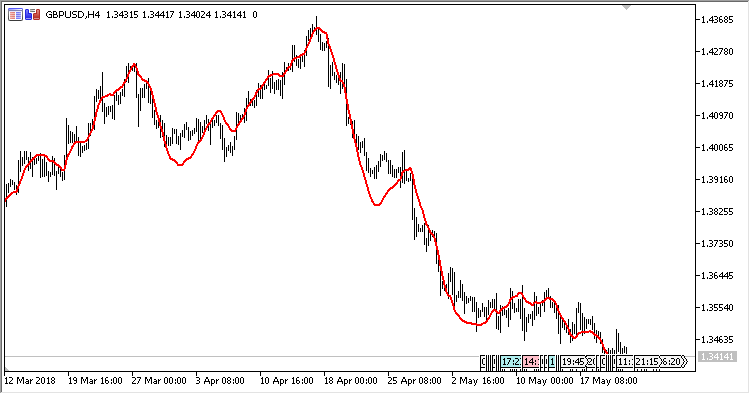# HWMA – indicator for MetaTrader 5

Indicator HWMA (Holt-Winter Moving Average) is a three-parameter moving average by the Holt-Winter method; the three parameters should be selected to obtain a forecast.

The indicator has four input parameters:

• nA – parameter of the equation that describes a smoothed series (from 0 to 1);
• nB – parameter of the equation to assess the trend (from 0 to 1);
• nC – parameter of the equation to assess seasonality (from 0 to 1);
• Applied price – price used for calculations.
Related Posts

Calculations:

`HWMA[i] = F[i] + V[i] + 0.5 * A[i]`

where:

```F[i] = (1-nA) * (F[i-1] + V[i-1] + 0.5 * A[i-1]) + nA * Price[i]
V[i] = (1-nB) * (V[i-1] + A[i-1]) + nB * (F[i] - F[i-1])
A[i] = (1-nC) * A[i-1] + nC * (V[i] - V[i-1])
```